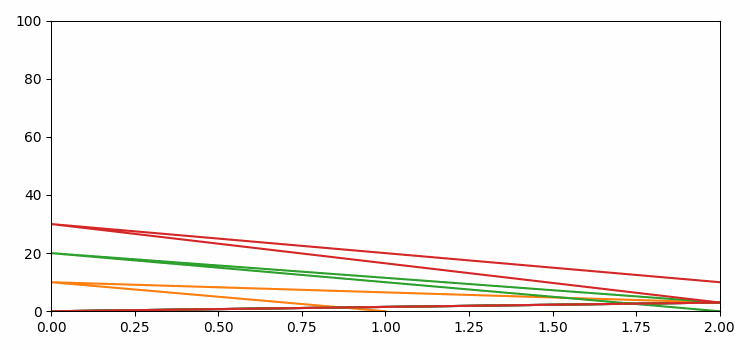# Defining multiple plots to be animated with a for loop in Matplotlib

To define multiple plots to be animated with a for loop in matplotlib, we can take followings steps −

• Set the figure size and adjust the padding between and around the subplots.
• Create a new figure or activate an existing figure using figure method.
• Add an axes to the current figure and make it the current axes.
• Initialize two variables, N and x, using numpy.
• Get the list of lines and bar patches.
• Animate the lines and rectangles (bar patches) in a for loop.
• Make an animation by repeatedly calling a function *func*.
• To display the figure, use show() method.

## Example

from matplotlib import pyplot as plt
from matplotlib import animation
import numpy as np

plt.rcParams["figure.figsize"] = [7.50, 3.50]
plt.rcParams["figure.autolayout"] = True

fig = plt.figure()
ax = plt.axes(xlim=(0, 2), ylim=(0, 100))
N = 4
x = np.linspace(-5, 5, 100)
lines = [plt.plot(x, np.sin(x)) for _ in range(N)]
rectangles = plt.bar([0.5, 1, 1.5], [50, 40, 90], width=0.1)
patches = lines + list(rectangles)

def animate(i):
for j, line in enumerate(lines):
line.set_data([0, 2, i, j], [0, 3, 10 * j, i])
for j, rectangle in enumerate(rectangles):
rectangle.set_height(i / (j + 1))
return patches

anim = animation.FuncAnimation(fig, animate,
frames=100, interval=20, blit=True)

plt.show()

## Output